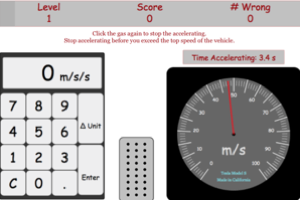Programs for 2nd Law (Tracking Single Particle)
Below are all the programs that might be used with this topic. Click on "See Resources" to see if there are any student directions, lab sheets or other materials that have been created for this program.
Equations of Motion Lab

Lab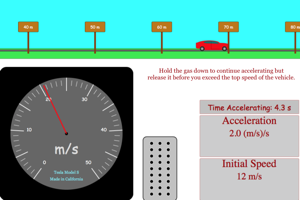Photogate Acceleration Prelab

LabNewton's 2nd Law with Photogates

LabFirework Vertical Motion Lab

Lab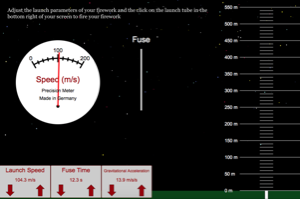Car Acceleration Program

HomeworkNewton's Law Simple

Homework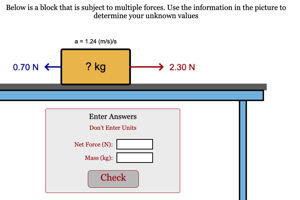Time to Traverse Table

Homework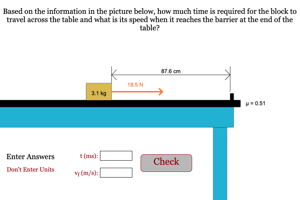Acceleration Challenge

Game